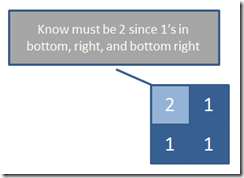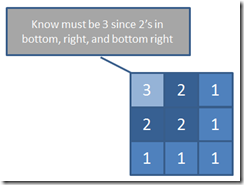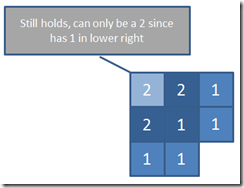# James Michael Hare

...hare-brained ideas from the realm of software development...

### News

Welcome to my blog! I'm a Sr. Software Development Engineer in the Seattle area, who has been performing C++/C#/Java development for over 20 years, but have definitely learned that there is always more to learn!

All thoughts and opinions expressed in my blog and my comments are my own and do not represent the thoughts of my employer.

## Solution to Little Puzzlers–Largest Square of ‘1’s in a Matrix

This is the way I went about the “Largest Square of '1's in a Matrix” problem. However, keep in mind there are multiple ways to solve this, so don't worry if your solution has variations and it’s entirely possible there are more efficient ways.

Feel free to suggest your solution in the comments here or in the original post, but please be respectful of others efforts.

### My Approach

This is one of the more fun problems I’ve ever had to tackle in an evaluation, and got to a fairly optimal solution with a wee bit of prompting.  It does sacrifice a bit of space for speed (though technically you don’t need the extra space if you’re free to destroy the matrix).

There is, of course, the naïve solution of starting at each location, and seeing if a square exists at that location.  Basically, you would go right until you encounter a zero, and this would be the proposed “size” of the square.  Then you’d try to go down a row and see if you can go that many to the right again.  if at any time you hit a zero faster than that, you’d shorten your “size” to that.  Repeat until you’ve checked “size” number of rows and then you know the size of the square at that point.

Unfortunately, that will get expensive for large matrices, and there’s a much more optimal way that you can solve this with only making one pass through the matrix, which I will describe now.

Basically, what we want to do is find the top-left of the square, so I’ll start at the bottom right of the matrix and look for squares based on the information from previous passes.

Of course, a lone ‘1’ is always at least a square of size ‘1’, so that’s not as interesting and fairly obvious.  The trick is to find squares of size 2 and above.

So consider a 2x2 grid of ‘1’s starting at (r,c):We know that the square at (r,c) must be at least size ‘2’ if it has a ‘1’ and there are squares of size ‘1’ at (r+1, c), (r, c+1), and (r+1,c+1).  This only makes sense, if (r,c) is one and there are squares of size ‘1’ bellow, to the right, and below and to the right, then we know we have a square of at least size ‘2’.

So what about size ‘3’?  Consider a 3x3 square of ‘1’s at (r,c):Again, we know that there must be a square of size ‘3’ at (r,c) if there are squares of size ‘2’ at (r+1, c), (r, c+1), and (r+1, c+1).  In fact, you can extend this to the generic logic:

size of square at (r,c) = min (size of squares at (r,c+1), (r+1, c), (r+1, c+1)) + 1

Why min?  The min covers the holes.  For example, if you were missing the bottom right ‘1’ above, you’d have this:Our logic still holds.  Notice that now, the middle square is only a square of size 1, because the minimum of it’s 3 squares to consider is 0 and 0 + 1 = 1.  This still makes a square of 2 at (r+1, c) and (r, c+1) since their 3 squares of consideration are all ‘1’s, and that ultimately makes (r,c) a ‘2’ since the minimum of it’s three squares under consideration is ‘1’ and 1 + 1 = 2.

Armed with this general knowledge, I can create a scratchpad of the same size as my matrix, and build up the size of the squares (as we did above) whenever I find a 1.  We’ll need to do a little ‘magic’ when we consider squares outside the matrix (on the lower row and rightmost column), but as long as we consider those to be zeros, our logic works.

When we complete one pass of this algorithm, we should know the largest square in our matrix!

### The Code

`   1: // class to analyze a matrix and find its largest square`
`   2: public class MatrixAnalyzer`
`   3: {`
`   4:     // gets the matrix to analyze`
`   5:     public int[,] Matrix { get; private set; }`
`   6:  `
`   7:     // gets the row count`
`   8:     public int Rows`
`   9:     {`
`  10:         get`
`  11:         {`
`  12:             return Matrix.GetLength(0);`
`  13:         }`
`  14:     }`
`  15:  `
`  16:     // gets the column count`
`  17:     public int Columns`
`  18:     {`
`  19:         get`
`  20:         {`
`  21:             return Matrix.GetLength(1);`
`  22:         }`
`  23:     }`
`  24:  `
`  25:     // initializes a new analyzer given a matrix`
`  26:     public MatrixAnalyzer(int[,] matrix)`
`  27:     {`
`  28:         if (matrix == null) throw new ArgumentNullException("matrix");`
`  29:  `
`  30:         Matrix = matrix;`
`  31:     }`
`  32:  `
`  33:     // walk through finding the largest square from the back of the matrix`
`  34:     // forward, using the scratchpad to build up our current sizes`
`  35:     public Tuple<Point, int> FindLargestSquare()`
`  36:     {`
`  37:         int maxRow = 0;`
`  38:         int maxCol = 0;`
`  39:  `
`  40:         var scratchPad = new int[Rows, Columns];`
`  41:  `
`  42:         for (int row = Rows - 1; row >= 0; --row)`
`  43:         {`
`  44:             for (int col = Columns - 1; col >= 0; --col)`
`  45:             {`
`  46:                 scratchPad[row, col] = (Matrix[row, col] == 1) ? GetRank(scratchPad, row, col) + 1 : 0;`
`  47:  `
`  48:                 if (scratchPad[row, col] > scratchPad[maxRow, maxCol])`
`  49:                 {`
`  50:                     maxRow = row;`
`  51:                     maxCol = col;`
`  52:                 }`
`  53:             }`
`  54:         }`
`  55:  `
`  56:         return Tuple.Create(new Point { X = maxRow, Y = maxCol }, scratchPad[maxRow, maxCol]);`
`  57:     }`
`  58:  `
`  59:     // helper method to get the rank of the scratchpad square considering the squares`
`  60:     // to the right, below, and right & below.`
`  61:     private int GetRank(int[,] scratchPad, int row, int col)`
`  62:     {`
`  63:         return Math.Min(`
`  64:             Math.Min(GetValue(scratchPad, row, col + 1), GetValue(scratchPad, row + 1, col)),`
`  65:             GetValue(scratchPad, row + 1, col + 1));`
`  66:     }`
`  67:  `
`  68:     // helper method to get value of scratch pad considering limits`
`  69:     private int GetValue(int[,] scratchPad, int row, int col)`
`  70:     {`
`  71:         return row < Rows && col < Columns ? scratchPad[row, col] : 0;`
`  72:     }`
`  73: }`

### Summary

Hope you had fun with this one!  Of course, I’m sure many out there can tweak the answer for performance in various ways – but you get the point.

Have a solution that worked for you but was totally different?  I’d love to hear about it!

Stay tuned next week for the next Little Puzzler.

Print | posted on Monday, April 27, 2015 10:24 PM |